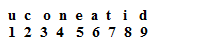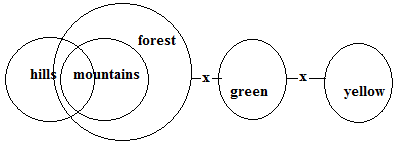# SSC CGL 2018 Practice Test Papers | Reasoning Ability (Day-8)

Dear Aspirants, Here we have given the Important SSC CGL Exam 2018 Practice Test Papers. Candidates those who are preparing for SSC CGL 2018 can practice these questions to get more confidence to Crack SSC CGL 2018 Examination.

[WpProQuiz 2736]

Click “Start Quiz” to attend these Questions and view Explanation

1. Choose the correct alternative for

27 : 128 :: 37 : ?

1. 2098
2. 2187
3. 356
4. 567
1. Harshita was 17th from left end of the row and Deepthi was 13th from right end of the row. How many persons are standing in the row if keerthi was standing 27th from both left and right end?
1. 42
2. 38
3. 51
4. 53
1. 17, 42, 7, 52, – 3,?
1. 58
2. 62
3. 33
4. 76
1. Unscramble the word1. 591267834
2. 123546897
3. 591762843
4. 785321469
1. PQ: TY::AB: ??
1. EJ
2. JE
3. FH
4. SY
1. A≥B≥C<Z≥P; T>S<P≤E

i)A>T

ii) E>A

1. Either i or ii follow
2. Neither i nor ii follow
3. Only i follows
4. Only ii follows
1. Statement: some hills are mountain.

All mountains are forest.

No forest is green.

No green is yellow.

Conclusion: (A) Some hills are not yellow

(B) All yellow is forest is a possibility

1. Only B follow
2. Only a follow
3. Neither A nor B follow
4. Both A and B follows
1. Which operation is correct?
1. 10 ÷ 4+ 24* 12- 4= 8
2. 10- 4+ 24 *12÷ 4 =54
3. 10 * 4 -24 ÷12 *4 =32
4. 10 + 4 -24* 12 ÷4 =20
1. Find the number to be filled in the table as per the logic followed.
 7 17 37 9 24 54 11 31 ?
1. 64
2. 71
3. 43
4. 76
1. Find the odd one
1. A5F
2. C9M
3. D13P
4. L8U

27=128; 37=2187

Keerthi is standing  in the middle of the row, No. of persons in the row= 26×2+1=53

Series is +25, -35, +45,-55+65…..

The hidden word is education-591267834

P+4; Q+8=TY

A+4: B+8=EJ7+10=17; 17+20=37

9+15=24; 24+30=54

11+20=31; 31+40=71

No. of letters between A and F=5

No. of letters between C and M=9

No. of letters between D and P=11

No. of letters between L and U=8